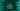# 3 different C programs to convert kilometers to miles## C program to convert kilometers to miles:

In this post, we will learn how to convert kilometers to miles in C programming. If we know the kilometer value, we can convert it easily to miles. This is actually easy if you know the formula to use for the conversion.

The program will take the kilometer value as an input from the user, it will convert it to miles, and print it out.

### How to convert Kilometers to Miles:

1 km is equal to 0.62137119 miles. So, if we get the kilometer value as an input from the user, we can multiply this value with 0.62137119 to get the value of the miles.

So, we can simply multiply this value with the kilometers value to get the required miles.

Let’s write it down in code.

### Method 1: C program to convert kilometers to miles with user input values:

The below C program takes the kilometer value as an input from the user, calculates the miles value, and prints it out.

``````#include <stdio.h>

int main()
{
float km, miles;

printf("Enter the Kilometer value: ");
scanf("%f", &km);

miles = km * 0.62137119;

printf("%.2fkm = %.2fmiles\n", km, miles);
}``````
• In this program, km and miles are two float variables to hold the kilometer and miles value.
• It asks the user to enter the kilometer value. It uses scanf to read the user-entered value and store it in the km variable.
• The miles value is calculated by multiplying the kilometer by 0.62137119 as explained before. This value is assigned to the miles variable.
• The last line is printing the final conversion result.

If you run this program, it will print output as below:

``````Enter the Kilometer value: 10
10.00km = 6.21miles

Enter the Kilometer value: 15
15.00km = 9.32miles``````

### Method 2: C program to convert kilometers to miles with macro:

Let’s use macro to make the above program more readable. If someone else reads the code, it will be hard to understand what the number 0.62137119 refers to. Instead, we can use a macro to hold this number and we can use that macro in the program:

``````#include <stdio.h>
#define ONE_KM_IN_MILES 0.62137119

int main()
{
float km, miles;

printf("Enter the Kilometer value: ");
scanf("%f", &km);

miles = km * ONE_KM_IN_MILES;

printf("%.2fkm = %.2fmiles\n", km, miles);
}``````

We created a new macro ONEKMIN_MILES that holds the number 0.62137119. It is now more readable than the previous example.

It will print similar results.

### Method 3: C program to convert kilometers to miles by using a separate function:

Let’s use a separate function to convert a kilometer value to miles. This method will be called from main and as this is a different function, we can call it from any other place if we want.

``````#include <stdio.h>
#define ONE_KM_IN_MILES 0.62137119

float kmToMiles(float km)
{
return km * ONE_KM_IN_MILES;
}

int main()
{
float km, miles;

printf("Enter the Kilometer value: ");
scanf("%f", &km);

miles = kmToMiles(km);

printf("%.2fkm = %.2fmiles\n", km, miles);
}``````

The kmToMiles method takes the kilometer value as its parameter and it returns the converted miles value. We are calling this method in main() and the result or returned value is stored in the miles variable.

You will get a similar result if you run this program.